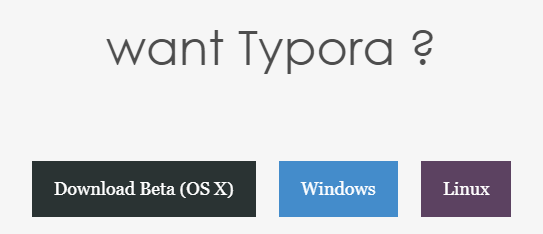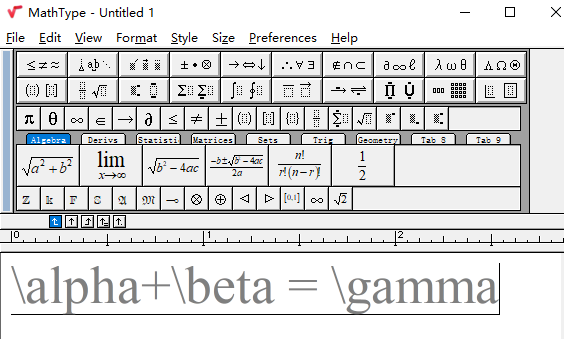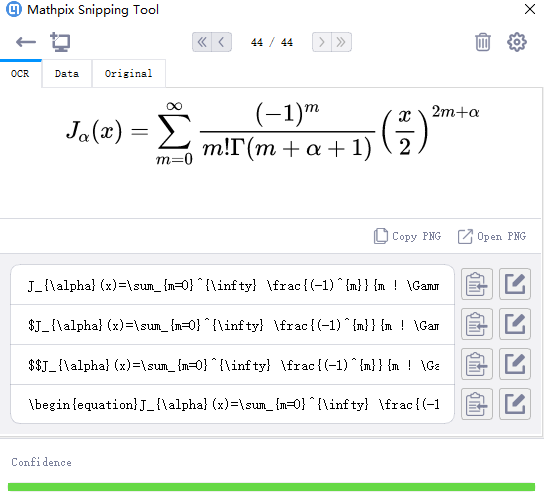### 1 Typora

#### 1.1 软件安装#### 1.2 Markdown与Latex 基本语法

##### 1.2.1 文字排版

1<center> 我是居中文字</center>

xxxxxxxxxx11<font size = 4 color = red>月来客栈</font>

##### 1.2.2 代码排版

xxxxxxxxxx21import sys2printf("Hello world!")

##### 1.2.3 公式排版

xxxxxxxxxx11$$J_\alpha(x) = \sum_{m=0}^\infty \frac{(-1)^m}{m! \Gamma (m + \alpha + 1)} {\left({ \frac{x}{2} }\right)}^{2m + \alpha} \text {，独立公式示例}$$

xxxxxxxxxx11f(x) = \sum_{i=1}^nx_i^2\tag{1}

xxxxxxxxxx41\begin{aligned}2f(x)&=\sum_{i=1}^nx_iw_i\\[2ex]3f(y)&=a+b+c+d+e+f4\end{aligned}

### 2 Mathpix Snipping Tool#### 2.1 安装与使用### 引用

 常用LaTex语法：https://www.zybuluo.com/codeep/note/163962